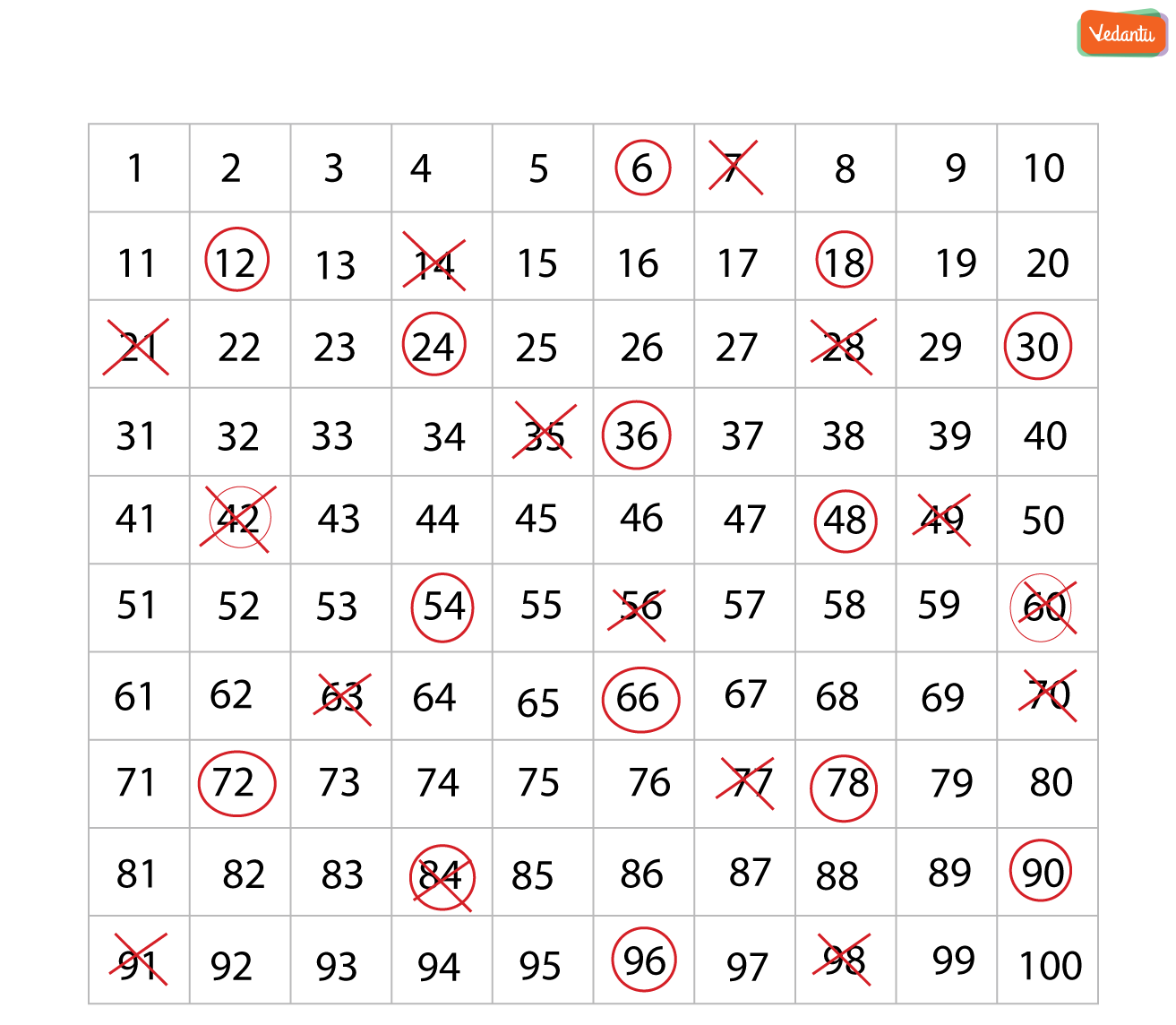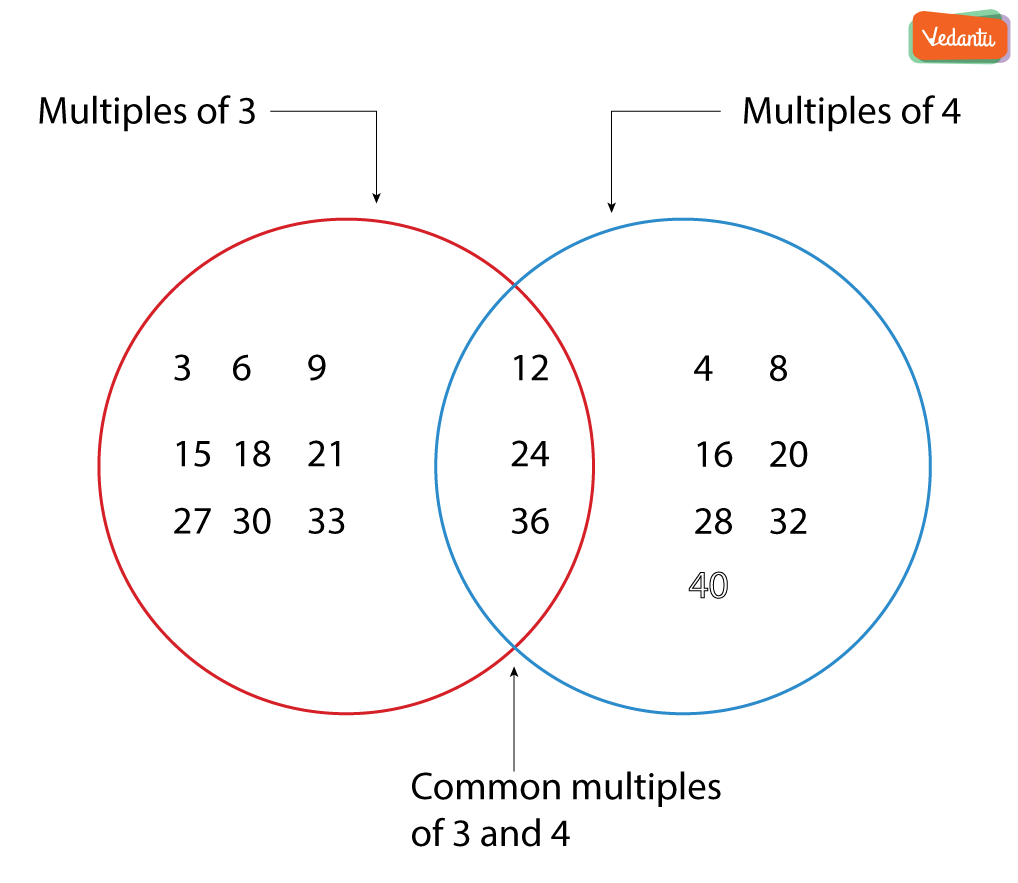Courses
Courses for Kids
Free study material
Offline Centres
More

# Common MultipleLast updated date: 22nd Nov 2023
Total views: 299.7k
Views today: 4.99k## Introduction to Common Multiple

Multiples are used in whole mathematics. The extensive use of multiples is because of getting commons. It is used in calculation to simplify a fraction. The fundamental principle of arithmetic is also telling us about the multiples only.

## Multiples

Multiples are numbers which we’ll get after multiplying a number with counting numbers. Let say we have a number n and we know that counting numbers are 1, 2, 3, 4, 5, 6…. Then, the product of n and 1, 2, 3, 4, 5, 6.. Is called multiples and this scenario is called multiplication.

Example: Let say we have a number 5. For getting it’s multiples we need to multiply it by counting numbers that are multiplication with 1, 2, 3, and so on. We’ll get

5 x 1 = 5

5 x 2 = 10

5 x 3 = 15

5 x 4 = 20

5 x 5 = 25

5 x 6 = 30

Hence, we’ll get multiples of 5 as 5, 10, 15, 20, 25 and so on.

## Common Multiples

Whenever we want to talk about the common immediately, more than one quantity comes in our mind. Hence, we’ll start with 2 numbers whose multiples we have. Let say we have numbers as 5 and 6. First of all we’ll find there multiples.

Multiples of 5 will be 5, 10 ,15, 20, 25, 30, 35, 40, and so on.

Multiples of 6 will be 6, 12, 18, 24, 30, 36, 42, 48, 54, 60 and so on.

Now to get common multiples we just need to find common numbers in both the multiples. Observe that in both the multiples set 30 is common. So, we’ll say 30 is the first common multiples or Least common multiple of 5 and 6. Least common multiple is commonly called LCM.

Now the obvious question comes in our mind, is there any multiple which is not least. The answer is yes. There are many common multiples after first or least. For example observe that for 5 and 6 first common multiples is 30 then second will be 60 third will be 90 and so on.

## Representation of Common Multiples

### Grid Method

Common multiples are the numbers which come common in both the multiples table of given numbers. If we take number 6 and 7 and we have to find the common multiples of them one way of representing them is following:We have marked the circle for all the multiples of 6 and cross for all the multiples of 7 till 100 numbers. Now what will be our common multiples?

Common multiples will simply be those numbers that are crossed as well as circled. That’s how they’ll come in both the multiple tables.

Hence, 42, 84 and so on will be our common multiples. Also, the least common multiple will be 42.

### Vann Diagram

Another way to represent common multiples is the Vann diagram. Suppose we have to find the common multiples of 3 and 4. Then we’ll close all the multiples of 3 and 4 in a circle separately. Then we’ll find commons in them. Kindly refer the following:### Solved Examples

1. Write the common multiples of 7 and 9.

Ans: First of all we’ll find the multiple of 7 which are 7, 14, 21, 28, 35, 42, 49, 56, 63, 70. Now, multiples of 9 are 9, 18, 27, 36, 45, 54, 63, 72, 90. Now the Common multiples of 7 and 9 will be the number that occurs in both the tables. Which are 63, 126, 189, and so on.

2. A and B are two numbers such that B is a multiple of A. Which of the following is the value of LCM (A,B)?

Ans: We have given two numbers A and B. For finding the least common multiple first we need to find their common multiples. Then we can find least out of them. We have also given that B is a multiple of A means when we’ll be writing multiples of A then B will occur in its table. Also, all the common multiples will be the multiples of B. Hence the LCM(A, B) will be B.

### Did You Know?

1. There are infinite common multiples possible for any two finite real numbers. We just have to write multiples of LCM.

2. The smallest common multiple is known as LCM. Which refers to least common multiple. The representation is LCM(a, b) which represents the least common multiple of a and b.

## FAQs on Common Multiple

1. How can we Find LCM of Two Numbers?

Ans: There are 3 methods to find common multiples.

• LCM by listing method.

• LCM by prime factorization.

• LCM by division method.

2. What are the Properties of Common Multiple?

Ans: Common multiples follow:

• Associative property

• Commutative property

• Distributive property